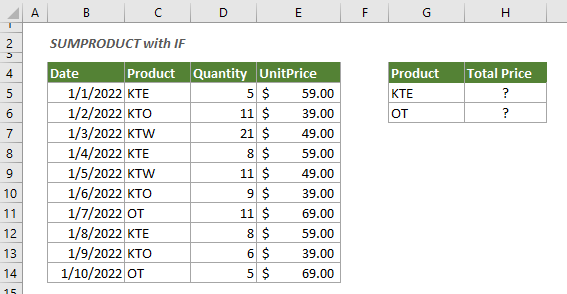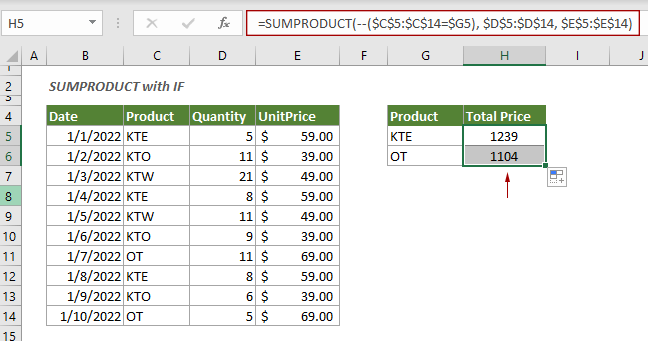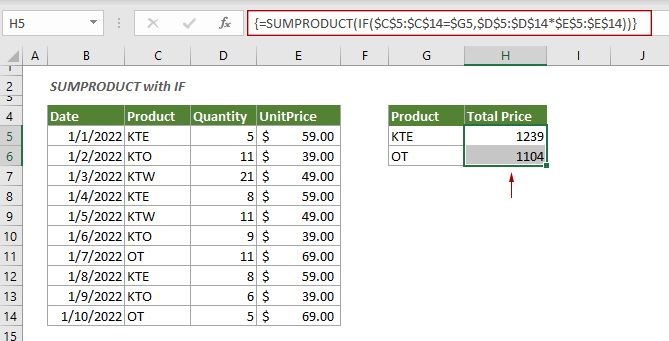## Use SUMPRODUCT with IF function in Excel

This tutorial is talking about how to use IF logic with SUMPRODUCT function in Excel.

#### How to use SUMPRODUCT with IF function in Excel?

Have a look at the following example. To sum the total price for the product “KTE” or “OT”, you can try the following formula.Generic Formulas

=SUMPRODUCT(--( array1=”condition”) , [array2] , [array3])

Arguments

Array1: The range of cells where the condition meet;
Condition: The condition defines which values in the Array1 will be multiplied then summed;
[Array2], [Array3]: These two arrays contain the values you will multiply then add based on the corresponding in Array1.

How to use this formula?

Select a blank cell next to the first condition “KTE” in G5, enter the formula below and press the Enter key to get the total price of “KTE”. Select this result cell and then drag its AutoFill Handle down to get the total price of “OT”.

=SUMPRODUCT(--(\$C\$5:\$C\$14=\$G5), \$D\$5:\$D\$14, \$E\$5:\$E\$14)Note: You can also nest the IF function inside of the SUMPRODUCT function to get it done:

=SUMPRODUCT(IF(\$C\$5:\$C\$14=\$G5,\$D\$5:\$D\$14*\$E\$5:\$E\$14))

After entering the formula, press the Ctrl + Shift + Enter keys to confirm it.How does the formula work?

=SUMPRODUCT(--(\$C\$5:\$C\$14=\$G5), \$D\$5:\$D\$14, \$E\$5:\$E\$14)

\$C\$5:\$C\$14=\$G5: Here checks if the value in each cell of range C5:C14 equals to “KTE” (locates in G5). And returns an array like this: {TRUE;FALSE;FALSE;TRUE;FALSE;FALSE;FALSE;TRUE;FALSE;FALSE};
--{TRUE;FALSE;FALSE;TRUE;FALSE;FALSE;FALSE;TRUE;FALSE;FALSE}: These two minus signs convert “TRUE” into 1 and “FALSE” into 0. Here the array turns to {1;0;0;1;0;0;0;1;0;0}:
=SUMPRODUCT({1;0;0;1;0;0;0;1;0;0}, \$D\$5:\$D\$14, \$E\$5:\$E\$14): Then the SUMPRODUCT function returns the sum of the products of corresponding values (the number 1 in the array). Finally gets the result as 1239.

#### Related functions

Excel SUMPRODUCT function
The Excel SUMPRODUCT function can be used to multiply two or more columns or arrays together, and then get the sum of products.

#### Related formulas

Sum only visible cells or rows in a filtered list
This tutorial demonstrates a formula based on the SUBTOTAL function with a specified function number to help you sum only visible cells in a filtered list in Excel.

Sum values in horizontal range in Excel
To sum numbers in horizontal range based on a condition, you can apply the SUMIFS function. This step-by-step guide helps you to get it done.

Use SUMIFS with multiple criteria based on OR logic
By default, the SUMIFS function handles multiple criteria based on AND logic. If you want to sum multiple criteria based on OR logic, you need to use the SUMIFS function within an array constant.

### The Best Office Productivity Tools

#### Kutools for Excel - Helps You To Stand Out From Crowd

 Popular Features: Find, Highlight or Identify Duplicates  |  Delete Blank Rows  |  Combine Columns or Cells without Losing Data  |  Round without Formula ... Super VLookup: Multiple Criteria  |  Multiple Value  |  Across Multi-Sheets  |  Fuzzy Lookup... Adv. Drop-down List: Easy Drop Down List  |  Dependent Drop Down List  |  Multi-select Drop Down List... Column Manager: Add a Specific Number of Columns  |  Move Columns  |  Toggle Visibility Status of Hidden Columns  |  Compare Columns to Select Same & Different Cells ... Featured Features: Grid Focus  |  Design View  |  Big Formula Bar  |  Workbook & Sheet Manager | Resource Library (Auto Text)  |  Date Picker  |  Combine Worksheets  |  Encrypt/Decrypt Cells  |  Send Emails by List  |  Super Filter  |  Special Filter (filter bold/italic/strikethrough...) ... Top 15 Toolsets:  12 Text Tools (Add Text, Remove Characters ...)  |  50+ Chart Types (Gantt Chart ...)  |  40+ Practical Formulas (Calculate age based on birthday ...)  |  19 Insertion Tools (Insert QR Code, Insert Picture from Path ...)  |  12 Conversion Tools (Numbers to Words, Currency Conversion ...)  |  7 Merge & Split Tools (Advanced Combine Rows, Split Excel Cells ...)  |  ... and more

Kutools for Excel Boasts Over 300 Features, Ensuring That What You Need is Just A Click Away...#### Office Tab - Enable Tabbed Reading and Editing in Microsoft Office (include Excel)

• One second to switch between dozens of open documents!
• Reduce hundreds of mouse clicks for you every day, say goodbye to mouse hand.
• Increases your productivity by 50% when viewing and editing multiple documents.
• Brings Efficient Tabs to Office (include Excel), Just Like Chrome, Edge and Firefox.